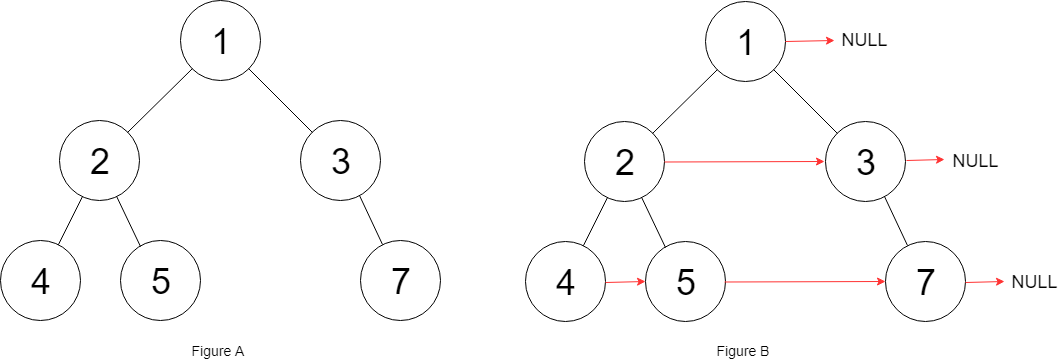## Algorithm

Problem Nmae: 117. Populating Next Right Pointers in Each Node II

Given a binary tree

```struct Node {
int val;
Node *left;
Node *right;
Node *next;
}
```

Populate each next pointer to point to its next right node. If there is no next right node, the next pointer should be set to `NULL`.

Initially, all next pointers are set to `NULL`.

Example 1:```Input: root = [1,2,3,4,5,null,7]
Output: [1,#,2,3,#,4,5,7,#]
Explanation: Given the above binary tree (Figure A), your function should populate each next pointer to point to its next right node, just like in Figure B. The serialized output is in level order as connected by the next pointers, with '#' signifying the end of each level.
```

Example 2:

```Input: root = []
Output: []
```

Constraints:

• The number of nodes in the tree is in the range `[0, 6000]`.
• `-100 <= Node.val <= 100`

## Code Examples

### #1 Code Example with C Programming

```Code - C Programming```

``````
struct TreeLinkNode *v, *h, *prev, *left;
prev = NULL;
left = root;
v = left;
while (v) {
h = v;
do {
if (h->left) {
if (prev) {
prev->next = h->left;
} else {
left = h->left;
}
prev = h->left;
}
if (h->right) {
if (prev) {
prev->next = h->right;
} else {
left = h->right;
}
prev = h->right;
}
h = h->next;
} while (h);
v = left;
prev = left = NULL;
}
}
``````
Copy The Code &

Input

cmd
root = [1,2,3,4,5,null,7]

Output

cmd
[1,#,2,3,#,4,5,7,#]

### #2 Code Example with C++ Programming

```Code - C++ Programming```

``````
class Solution {
public:
if(!root) return;
dequecur;
dequenext;
cur.push_back(root);
while(!cur.empty()){
cur.pop_front();
node->next = cur.empty() ? NULL : cur.front();
if(node->left) next.push_back(node->left);
if(node->right) next.push_back(node->right);
if(cur.empty()) swap(cur, next);
}
}
};
``````
Copy The Code &

Input

cmd
root = [1,2,3,4,5,null,7]

Output

cmd
[1,#,2,3,#,4,5,7,#]

### #3 Code Example with Java Programming

```Code - Java Programming```

``````
class Solution {
public Node connect(Node root) {
while (queue.peek() != null) {
int size = queue.size() - 1;
while (size-- > 0) {
Node removed = queue.remove();
removed.next = queue.peek();
if (removed.left != null) {
}
if (removed.right != null) {
}
}
queue.remove();
}
return root;
}
}
``````
Copy The Code &

Input

cmd
root = []

Output

cmd
[]

### #4 Code Example with Javascript Programming

```Code - Javascript Programming```

``````
const connect = function(root) {
if (root == null) return null
const cur = [root]
while (cur.length) {
const len = cur.length
for (let i = 0; i < len; i++) {
const el = cur.shift()
if (i === len - 1) el.next = null
else el.next = cur
if (el.left) cur.push(el.left)
if (el.right) cur.push(el.right)
}
}
return root
}
``````
Copy The Code &

Input

cmd
root = []

Output

cmd
[]

### #5 Code Example with Python Programming

```Code - Python Programming```

``````
class Solution:
def connect(self, root: "Node") -> "Node":
dummy = Node(-1, None, None, None)
tmp = dummy
res = root
while root:
while root:
if root.left:
tmp.next = root.left
tmp = tmp.next
if root.right:
tmp.next = root.right
tmp = tmp.next
root = root.next
root = dummy.next
tmp = dummy
dummy.next = None

return res
``````
Copy The Code &

Input

cmd
root = [1,2,3,4,5,null,7]

Output

cmd
[1,#,2,3,#,4,5,7,#]

### #6 Code Example with C# Programming

```Code - C# Programming```

``````
namespace LeetCode
{
public class _0117_PopulatingNextRightPointersInEachNode2
{
{
while (cur != null)
{
while (cur != null)
{
if (cur.left != null)
{
if (nextPrevious != null)
nextPrevious.next = cur.left;
else
nextPrevious = cur.left;
}

if (cur.right != null)
{
if (nextPrevious != null)
nextPrevious.next = cur.right;
else
nextPrevious = cur.right;
}

cur = cur.next;
}

}

return root;
}
}
}
``````
Copy The Code &

Input

cmd
root = [1,2,3,4,5,null,7]

Output

cmd
[1,#,2,3,#,4,5,7,#]